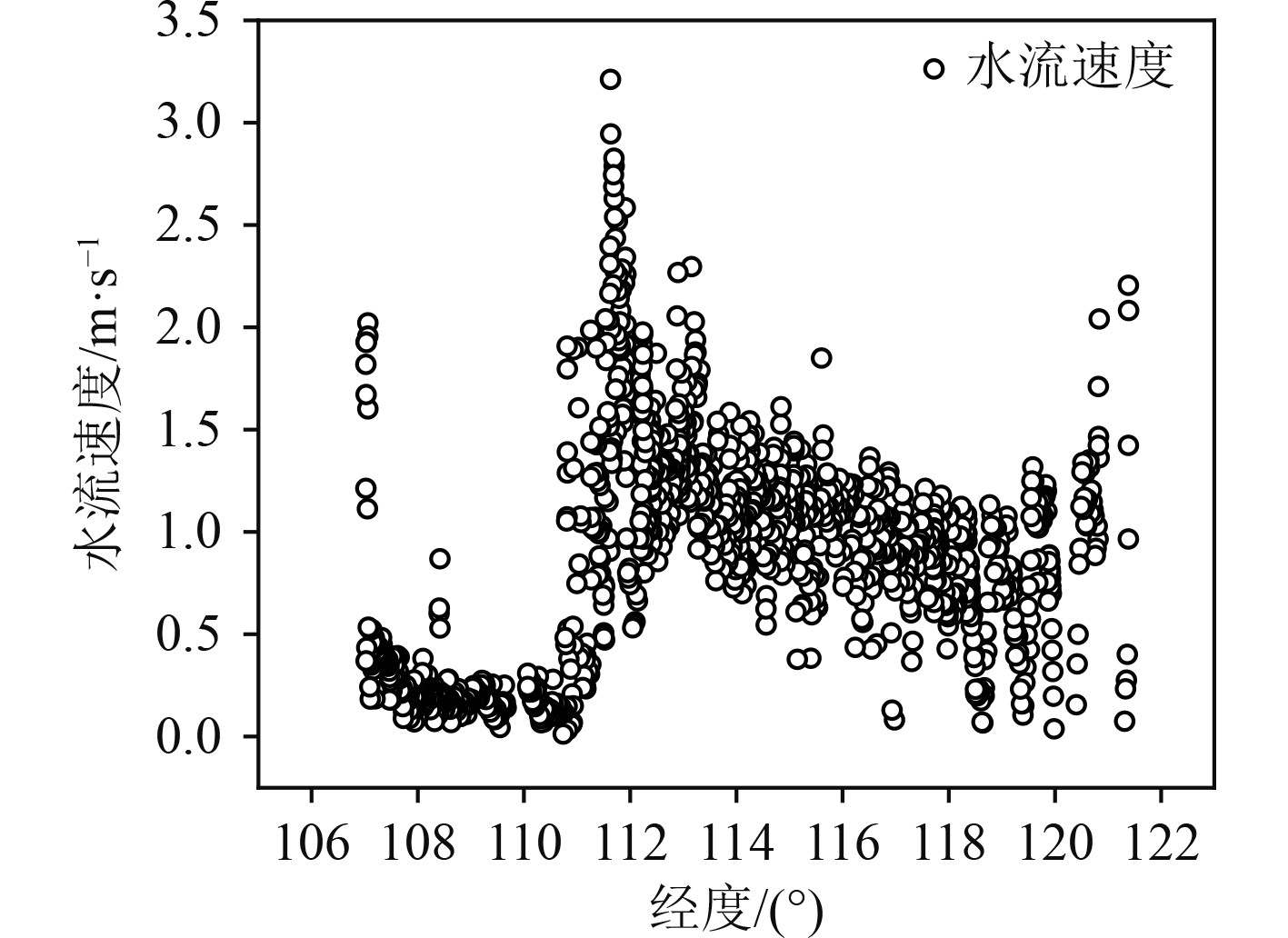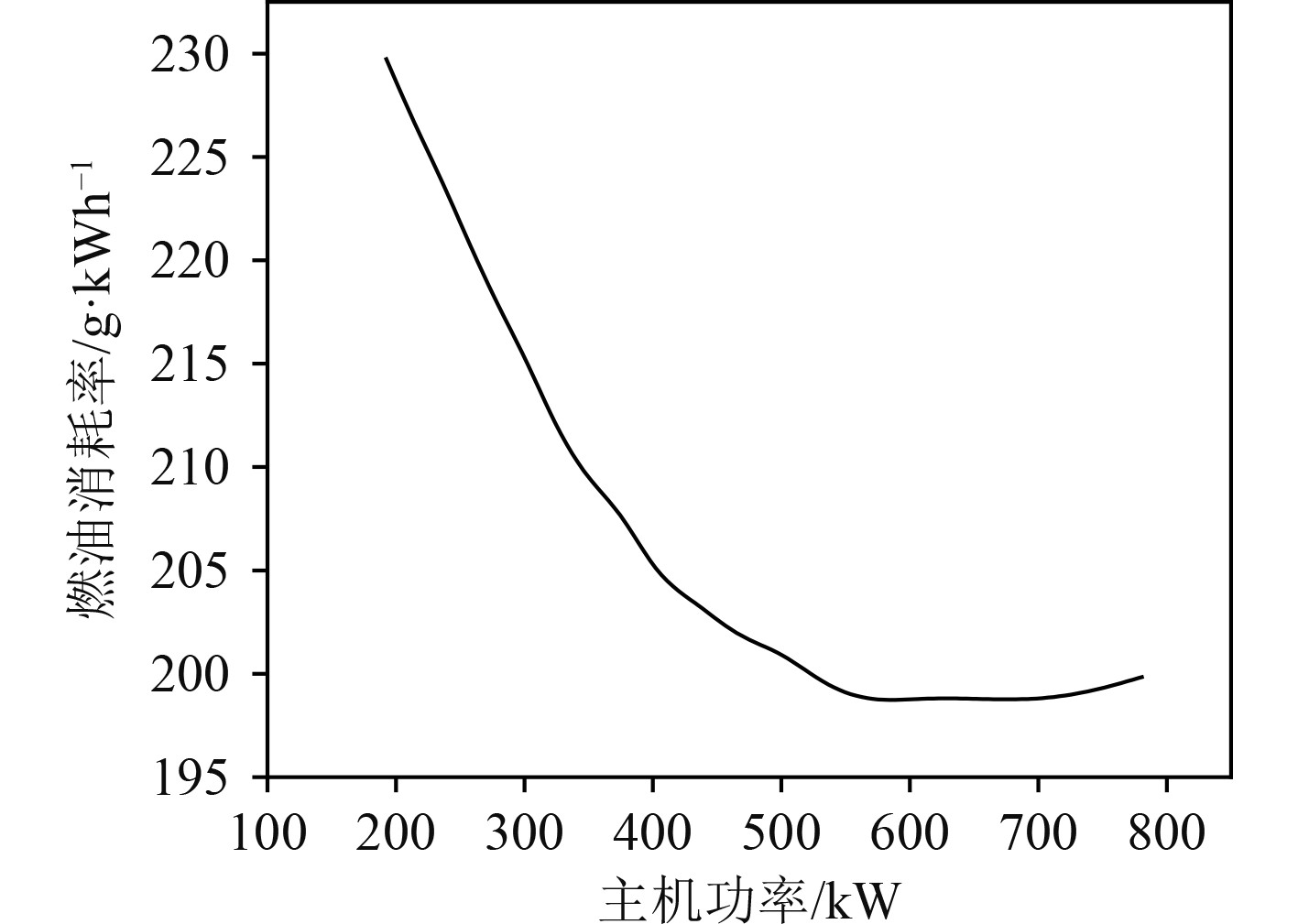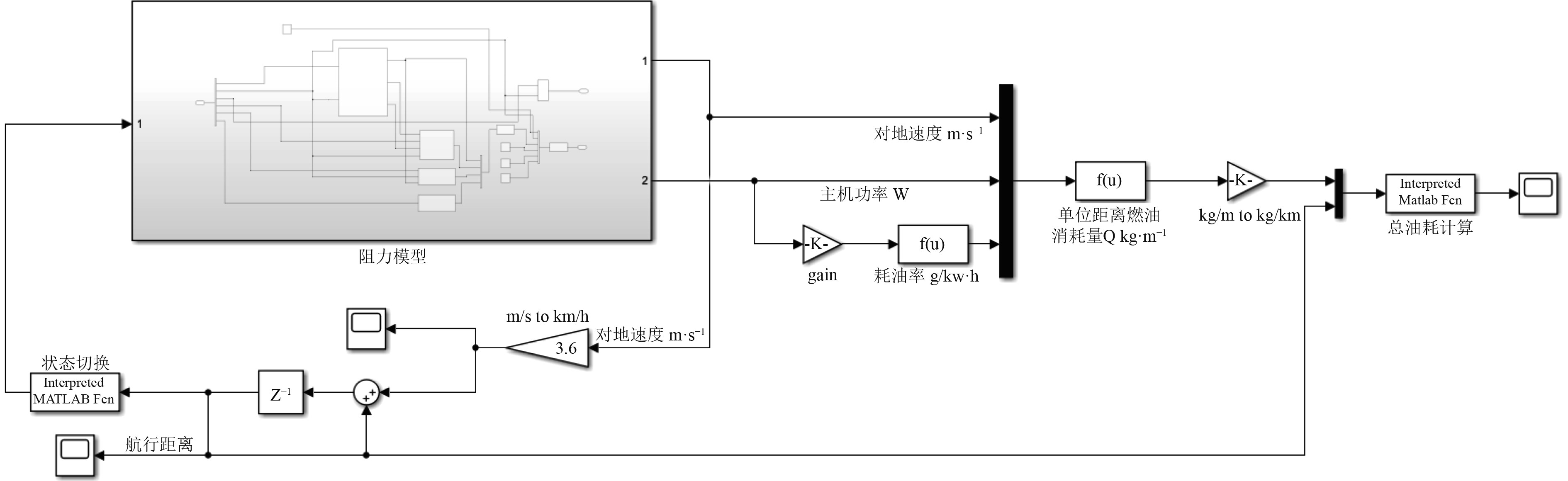﻿ 船舶能耗模型构建及清洁能源适用性分析
 舰船科学技术2023, Vol. 45Issue (1): 135-140    DOI: 10.3404/j.issn.1672-7649.2023.01.024PDF

1. 武汉理工大学 船海与能源动力工程学院，湖北 武汉 430063;
2. 中国船级社 武汉规范研究所，湖北 武汉 430022

Ship energy consumption model construction and clean energy applicability analysis
TAN Tao1, WANG Li-zheng1, ZHANG Wei2
1. School of Naval Architecture Ocean and Energy Power Engineering, Wuhan University of Technology, Wuhan 430063, China;
2. Wuhan Rules Research Institute, China Classification Society, Wuhan 430022, China
Abstract: Clean energy application is the main measure to achieve the carbon reduction target in shipping industry. The operating characteristics of clean energy powered ships are more related to the navigational environment than diesel powered ships, and accurate calculation of energy consumption and emission of ships is the prerequisite for clean energy applicability analysis. Matlab/Simulink platform is used to construct an energy consumption calculation model based on the navigable environment and actual operation strategy. Taking the Yangtze River 7 500-ton dry bulk carrier as an example, the calculation results of different energy power forms are analyzed comprehensively, and the suitable clean energy application scheme is recommended. The results show that LNG is the applicable clean energy source at the current stage, and hybrid power and diesel-LNG dual-fuel mechanical power are the suitable power forms.
Key words: clean energy     navigable environment     operation strategy     energy consumption models
0 引　言

1 船舶清洁能源应用现状

1.1 LNG动力船

1.2 甲醇动力船

2016年，Waterfront航运公司租赁的3艘5万吨甲醇运输船将成为全球首批使用甲醇作为燃料的远洋运输船。Proman Stena Bulk在广船国际订造2艘甲醇动力49900载重吨油船。甲醇正在成为最可行、排放量最低且具有成本效益的船用燃料替代品之一。与常规船用燃料相比，甲醇可减少95%以上的硫氧化物、颗粒物和60%的氮氧化物。除清洁环保外，由于甲醇是一种安全、可降解的船用燃料，甲醇动力船的改造费用更低。甲醇为未来船舶减碳提供了一条途径，但目前长江船用甲醇供应链处于空白，短期内不能应用于长江船舶。

1.3 纯电池动力船

1.4 燃料电池动力船

1.5 太阳能动力船

1.6 风能动力船

2 基于通航环境特征和营运工况的船舶能耗模型构建

2.1 能耗理论模型

 ${Q_{}} = \sum\limits_{b = 1}^m {} \sum\limits_{a = 1}^n {\left[ {c \cdot {G_{b,a}} \cdot {D_a}} \right]} + {Q_{aux}} 。$ (1)

 ${G_{b,a}} = \frac{{{P_{b,a}} \cdot {g_{b,a}}}}{{{v_{g,b,a}}}}。$ (2)

 ${P_{b,a}} = \frac{{{R_{b,a}} \cdot {V_{S,b,a}}}}{{K \cdot {\eta _S} \cdot {\eta _G} \cdot {\eta _R} \cdot {\eta _H} \cdot {\eta _0}}} 。$ (3)

 ${V_{S,b,a}} = {v_{g,b,a}} \pm {v_{w,g,a}}。$ (4)

 $\begin{split} {R_{b,a}} & = {R_{T,b,a}} + {R_{wave,b,a}} + {R_{wind,b,a}} + {R_{shallow,b,a}}= \\ & F\left( {{v_{g,b,a}},{v_{w,b,a}},{v_{wind,b,a}},{H_{b,a}}} \right) 。\end{split}$ (5)

2.2 航段划分

2.3 能耗模型主要影响参量确定

2.3.1 船舶对地航速表 1 船舶对地航速 Tab.1 Ship to ground speed
2.3.2 通航环境表 2 各航段航道维护水深 Tab.2 Depth of waterway maintenance for each navigation section表 3 部分实测有效数据 Tab.3 Part of the measured valid data图 1 水流分布情况 Fig. 1 Distribution of water flow表 4 各航段平均水流速度和风速 Tab.4 Average current speed and wind speed for each navigation section
2.3.3 燃油消耗率图 2 燃油消耗率系数图 Fig. 2 Fuel consumption rate coefficient chart图 3 主机能耗模型 Fig. 3 Host energy model
2.5 模型验证表 5 采集数据与仿真结果对比 Tab.5 Comparison of acquisition data and simulation results

3 长江散货船清洁能源适用性分析 3.1 分析对象及能源应用方案设定表 6 动力选型参数表 Tab.6 Power selection parameters table
3.2 方案比选

 $J{\text{ = }}\alpha {f_1}\left( x \right) + \beta {f_2}\left( x \right) 。$ (6)

1）必要货运费率 ${f_{_1}}\left( x \right)$ 是表征船舶在营运过程中单位运量成本的指标，航运公司对于成本控制十分重视，该指标是船东造船考虑的关键要素。其表达式为：

 $RFR = \left( {\left( {P - L} \right) \times \left( {A/P,i,N} \right) + L \times i + Y} \right) \times {10^{ - 4}}/Q 。$ (7)

2）船舶能效营运指数 ${f_2}\left( x \right)$ 是船舶营运过程中二氧化碳总量与货周转量的比值，即船舶运输单位货物、单位距离所产生的二氧化碳排放量。其表达式为：

 $EEOI = \frac{{\sum\limits_{j = 1}^x {F{C_j} \times {C_{Fj}}} }}{{{m_{c\arg o}} \times D}}。$ (8)表 7 评价函数及综合指标结果 Tab.7 Evaluation function and composite index results

4 结　语

  王思佳. 双碳目标下的航运减排路线图——访中国科学院院士、清华大学车辆与运载学院欧阳明高教授[J]. 中国船检, 2021(7): 46–50.  罗肖锋, 吴顺平, 雷伟, 等. 船舶能源低碳发展趋势及路径[J]. 中国远洋海运, 2021(3): 46-51.  SUN Xing, YAN Xinping, WU Bing, et al. Analysis on the operation energy efficiency for inland river ships[J]. Transportation Research Part D: Transport and Environment, 2013, 22: 34–39.  范爱龙, 严新平, 尹奇志, 等. 船舶主机能效模型[J]. 交通运输工程学报, 2015, 15(4): 69–76.  尹奇志, 陈兴, 李猛, 等. 内河电力推进游轮能效建模研究[J]. 内燃机, 2020(6): 5–11.  JEON M, NOH Y, SHIN Y, et al. Prediction of ship fuel consumption by using an artificial neural network[J]. Journal of Mechanical Science and Technology, 2018, 32(12): 5785-5796. DOI:10.1007/s12206-018-1126-4  叶睿, 许劲松. 基于人工神经网络的船舶油耗模型[J]. 船舶工程, 2016, 38(3): 85–88.  龚芳海, 吴道君. 舰船能耗预测的云数据挖掘[J]. 舰船科学技术, 2018, 40(24): 37–39.  王凯, 徐浩, 黄连忠, 等. 基于机器学习的船舶能耗智能预测方法分析[J]. 船舶工程, 2020, 42(11): 87–93.  中国船级社. 船舶能量消耗分布与节能指南[S]. 2014.  金校宇. 锐意创新立潮头 风正扬帆破浪行[N]. 中国交通报, 2021-12-14(5).